# Gauss-Bonnet theorem

(diff) ← Older revision | Latest revision (diff) | Newer revision → (diff)

The total curvatureof a two-dimensional compact Riemannian manifold, closed or with boundary, and the rotationof its smooth boundary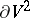are connected with the Euler characteristicof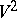by the relation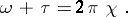Herewhereis the Gaussian curvature and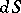is the area element;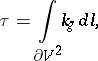whereis the geodesic curvature and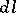is the line element of the boundary. The Gauss–Bonnet theorem is also valid for a manifold with a piecewise-smooth boundary; in that casewhereis the rotation of the boundary at a vertex. The theorem is valid, in particular, on regular surfaces in. The Gauss–Bonnet theorem was known to C.F. Gauss ; it was published by O. Bonnet  in a special form (for surfaces homeomorphic to a disc).

For a non-compact complete manifoldwithout boundary, an analogue of the Gauss–Bonnet theorem is the Cohn-Vossen inequality :The Gauss–Bonnet theorem and the given inequality are also valid for convex surfaces and two-dimensional manifolds of bounded curvature.

The Gauss–Bonnet theorem can be generalized to even-dimensional compact Riemannian manifolds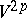, closed or with boundary: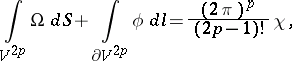where,denote the volume elements inand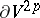, whileis some polynomial in the components of the curvature tensor of, andis some polynomial in the components of the curvature tensor and the coefficients of the second fundamental form of. The Gauss–Bonnet theorem has also been generalized to Riemannian polyhedra . Other generalizations of the theorem are connected with integral representations of characteristic classes by parameters of the Riemannian metric , , .